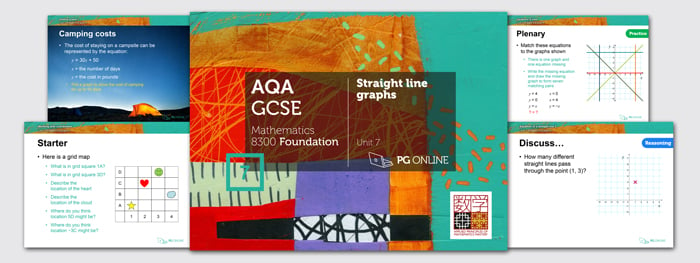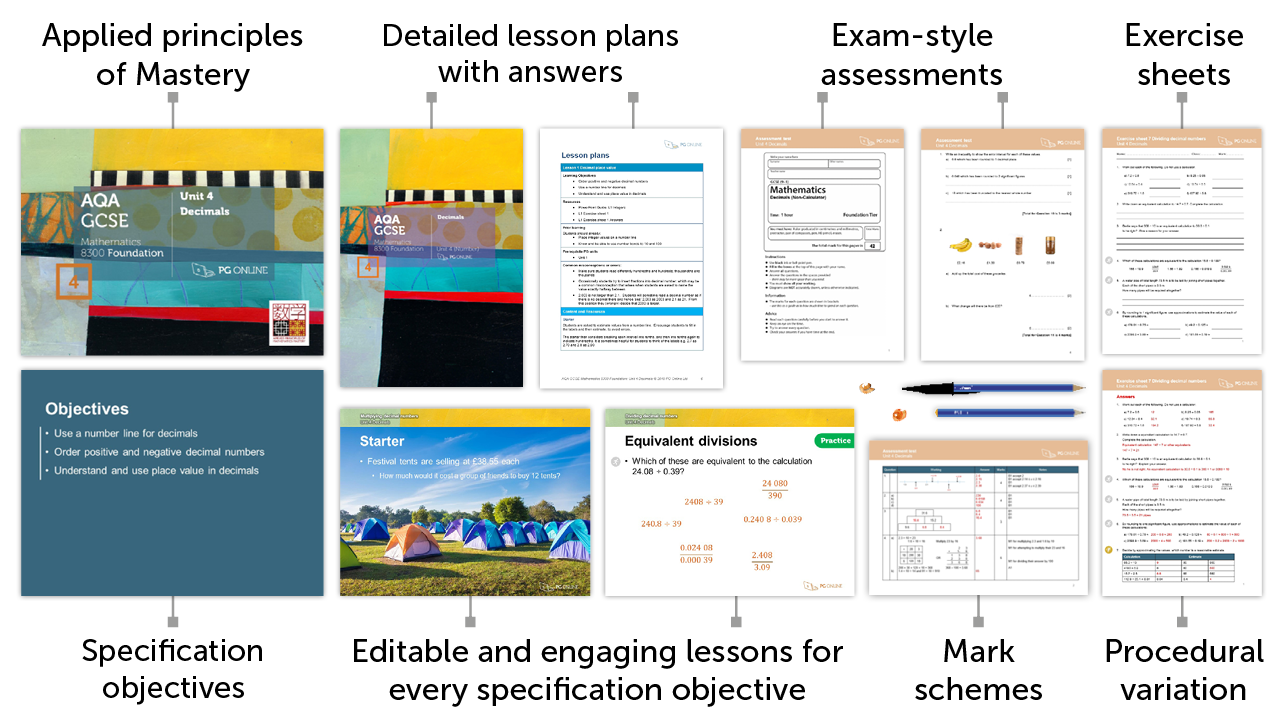# Mastery for GCSE AQA

### £75.00 + VATDiscounted rate from £15+

7

## Inside the Unit...### Areas Covered

• Lesson 1: Working with coordinates
• Lesson 2: Equations of lines
• Lesson 3: Plotting graphs 1
• Lesson 4: Plotting graphs 2
• Lesson 5: Gradients of straight lines
• Lesson 6: Equation of a straight line 1
• Lesson 7: Equation of a straight line 2
• Unit assessment

There are 7 exercise sheets and an examination-style assessment test, each with answers included in this unit.

## Mathematics Mastery

Our resources apply the principles of Mastery throughout, creating a rich and deep learning experience. Find out more about Mastery with our quick guide.## Summary

This unit considers the properties and uses of straight line graphs. With an emphasis on developing mastery, the unit begins by revisiting coordinates; reading and plotting values and finding the mid-point between two points. Equations of horizontal and vertical lines are explored and developed. Linear graphs are constructed from tables and an understanding of the form y = mx + c is developed and used to solve problems. The gradient and y-intercept of a straight line graph are explored, and skills developed so that students can plot a straight line without a table of values, from the gradient and y-intercept. Using context, the unit continues to develop skills in finding the equation of a line between two given points and a line with a given gradient that passes through a single given point. Reasoning problems are presented to support the development of students’ ability to apply their knowledge.

## What's included in the toolkit?The GCSE units have been written to satisfy the specification for the AQA 8300 Maths GCSE. Each unit contains:

• PowerPoint slides for each component
• Detailed lesson plans
• Learning objectives and outcomes
• Worksheets and homework activities with answers
• End-of-unit, exam-style assessment test with answers
• Other material and links to online resources

## What people say...

Lots of questions to gauge pupils understanding, great real life links with the slides, short pieces of info on each slide.

Abigail Alexander. Science teacher, Lutterworth College

I have already bought all of your Foundation resources and they are excellent!

Alistair Harris. Head of Maths, Kingstone High School

## Inside the Unit...

### Areas Covered

• Lesson 1: Working with coordinates
• Lesson 2: Equations of lines
• Lesson 3: Plotting graphs 1
• Lesson 4: Plotting graphs 2
• Lesson 5: Gradients of straight lines
• Lesson 6: Equation of a straight line 1
• Lesson 7: Equation of a straight line 2
• Unit assessment

There are 7 exercise sheets and an examination-style assessment test, each with answers included in this unit.

## Mathematics Mastery

Our resources apply the principles of Mastery throughout, creating a rich and deep learning experience. Find out more about Mastery with our quick guide.• ### How to order

1. Add individual units to a draft order or download a blank order form below to complete manually

2. Using a draft order you can either:

2. Email us your complete order
3. Create a PDF
(to fax or email at a later date)

• ### Unit Pricing and Discounts

Each unit is individually priced for LIFE. Please view each unit for detail.

Discounts are cumulative based on the total of units licenced to your department.

All prices subject to further discounts and VAT.

There are no annual renewal fees.

 2-5 units 10% discount 6-11 units 15% discount 12-23 units 20% discount 24+ units 25% discount